# Planar Graph in Graph Theory | Planar Graph Example

## Types of Graphs-

Before you go through this article, make sure that you have gone through the previous article on various Types of Graphs in Graph Theory.

We have discussed-

• A graph is a collection of vertices connected to each other through a set of edges.
• The study of graphs is known as Graph Theory.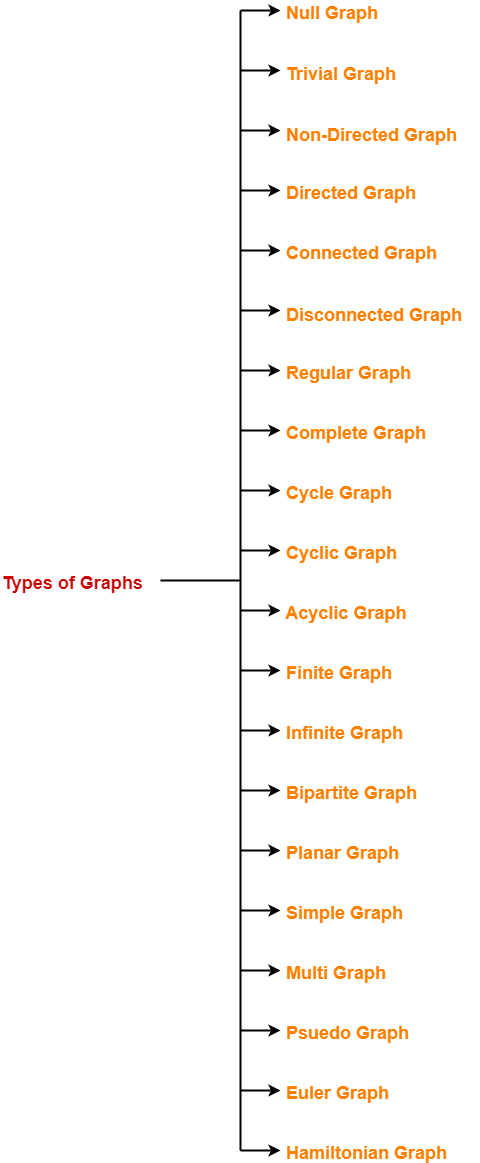## Planar Graph-

A planar graph may be defined as-

 In graph theory, Planar graph is a graph that can be drawn in a plane such that none of its edges cross each other.

### Planar Graph Example-

The following graph is an example of a planar graph-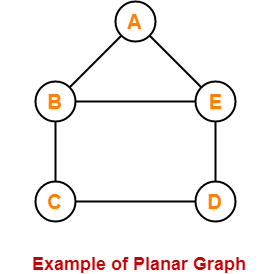Here,

• In this graph, no two edges cross each other.
• Therefore, it is a planar graph.

## Regions of Plane-

The planar representation of the graph splits the plane into connected areas called as Regions of the plane.

Each region has some degree associated with it given as-

• Degree of Interior region = Number of edges enclosing that region
• Degree of Exterior region = Number of edges exposed to that region

### Example-

Consider the following planar graph-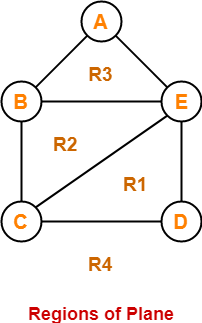Here, this planar graph splits the plane into 4 regions- R1, R2, R3 and R4 where-

• Degree (R1) = 3
• Degree (R2) = 3
• Degree (R3) = 3
• Degree (R4) = 5

## Planar Graph Chromatic Number-

• Chromatic Number of any planar graph is always less than or equal to 4.
• Thus, any planar graph always requires maximum 4 colors for coloring its vertices.

## Planar Graph Properties-

### Property-01:

In any planar graph, Sum of degrees of all the vertices = 2 x Total number of edges in the graph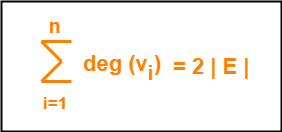### Property-02:

In any planar graph, Sum of degrees of all the regions = 2 x Total number of edges in the graph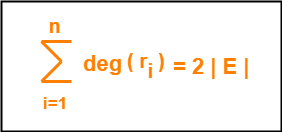### Case-01:

In any planar graph, if degree of each region is K, then-

 K x |R| = 2 x |E|

### Case-02:

In any planar graph, if degree of each region is at least K (>=K), then-

 K x |R| <= 2 x |E|

### Case-03:

In any planar graph, if degree of each region is at most K (<=K), then-

 K x |R| >= 2 x |E|

### Property-03:

If G is a connected planar simple graph with ‘e’ edges, ‘v’ vertices and ‘r’ number of regions in the planar representation of G, then-

 r = e – v + 2

This is known as Euler’s Formula.

It remains same in all the planar representations of the graph.

### Property-04:

If G is a planar graph with k components, then-

 r = e – v + (k + 1)

## Problem-01:

Let G be a connected planar simple graph with 25 vertices and 60 edges. Find the number of regions in G.

## Solution-

Given-

• Number of vertices (v) = 25
• Number of edges (e) = 60

By Euler’s formula, we know r = e – v + 2.

Substituting the values, we get-

Number of regions (r)

= 60 – 25 + 2

= 37

Thus, Total number of regions in G = 37.

## Problem-02:

Let G be a planar graph with 10 vertices, 3 components and 9 edges. Find the number of regions in G.

## Solution-

Given-

• Number of vertices (v) = 10
• Number of edges (e) = 9
• Number of components (k) = 3

By Euler’s formula, we know r = e – v + (k+1).

Substituting the values, we get-

Number of regions (r)

= 9 – 10 + (3+1)

= -1 + 4

= 3

Thus, Total number of regions in G = 3.

## Problem-03:

Let G be a connected planar simple graph with 20 vertices and degree of each vertex is 3. Find the number of regions in G.

## Solution-

Given-

• Number of vertices (v) = 20
• Degree of each vertex (d) = 3

### Calculating Total Number Of Edges (e)-

By sum of degrees of vertices theorem, we have-

Sum of degrees of all the vertices = 2 x Total number of edges

Number of vertices x Degree of each vertex = 2 x Total number of edges

20 x 3 = 2 x e

∴ e = 30

Thus, Total number of edges in G = 30.

### Calculating Total Number Of Regions (r)-

By Euler’s formula, we know r = e – v + 2.

Substituting the values, we get-

Number of regions (r)

= 30 – 20 + 2

= 12

Thus, Total number of regions in G = 12.

## Problem-04:

Let G be a connected planar simple graph with 35 regions, degree of each region is 6. Find the number of vertices in G.

## Solution-

Given-

• Number of regions (n) = 35
• Degree of each region (d) = 6

### Calculating Total Number Of Edges (e)-

By sum of degrees of regions theorem, we have-

Sum of degrees of all the regions = 2 x Total number of edges

Number of regions x Degree of each region = 2 x Total number of edges

35 x 6 = 2 x e

∴ e = 105

Thus, Total number of edges in G = 105.

### Calculating Total Number Of Vertices (v)-

By Euler’s formula, we know r = e – v + 2.

Substituting the values, we get-

35 = 105 – v + 2

∴ v = 72

Thus, Total number of vertices in G = 72.

## Problem-05:

Let G be a connected planar graph with 12 vertices, 30 edges and degree of each region is k. Find the value of k.

## Solution-

Given-

• Number of vertices (v) = 12
• Number of edges (e) = 30
• Degree of each region (d) = k

### Calculating Total Number Of Regions (r)-

By Euler’s formula, we know r = e – v + 2.

Substituting the values, we get-

Number of regions (r)

= 30 – 12 + 2

= 20

Thus, Total number of regions in G = 20.

### Calculating Value Of k-

By sum of degrees of regions theorem, we have-

Sum of degrees of all the regions = 2 x Total number of edges

Number of regions x Degree of each region = 2 x Total number of edges

20 x k = 2 x 30

∴ k = 3

Thus, Degree of each region in G = 3.

## Problem-06:

What is the maximum number of regions possible in a simple planar graph with 10 edges?

## Solution-

In a simple planar graph, degree of each region is >= 3.

So, we have 3 x |R| <= 2 x |E|.

Substituting the value |E| = 10, we get-

3 x |R| <= 2 x 10

|R| <= 6.67

|R| <= 6

Thus, Maximum number of regions in G = 6.

## Problem-07:

What is the minimum number of edges necessary in a simple planar graph with 15 regions?

## Solution-

In a simple planar graph, degree of each region is >= 3.

So, we have 3 x |R| <= 2 x |E|.

Substituting the value |R| = 15, we get-

3 x 15 <= 2 x |E|

|E| >= 22.5

|E| >= 23

Thus, Minimum number of edges required in G = 23.

To gain better understanding about Planar Graphs in Graph Theory,

Watch this Video Lecture

Next Article- Euler Graph

Get more notes and other study material of Graph Theory.

Watch video lectures by visiting our YouTube channel LearnVidFun.

SummaryArticle Name
Planar Graph in Graph Theory | Planar Graph Example
Description
Planar Graph in Graph Theory- A planar graph is a graph that can be drawn in a plane such that none of its edges cross each other. Planar Graph Example, Properties & Practice Problems are discussed.
Author
Publisher Name
Gate Vidyalay
Publisher Logo